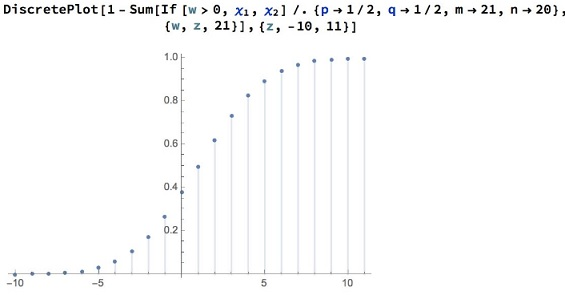# Coin Tossing Contest

### Problem### Solution 1

We shall consider several events concerning the possible total outcomes:

• $H_{B\gt A}$ - the event of $\mathcal{B}$ having more heads than $\mathcal{A},$
• $H_{B\le A}$ - the event of $\mathcal{B}$ having at most as few heads as $\mathcal{A},$
• $T_{B\gt A}$ - the event of $\mathcal{B}$ having more tails than $\mathcal{A},$

We are interested in the probability of the first of these events. Observe that $H_{B\le A}=T_{B\gt A}.$ On the other hand, when it comes to probabilities, then, by symmetry, $P(H_{B\gt A})=P(T_{B\gt A}).$

If $X$ is the total number of outcomes, then

$X=H_{B\gt A}\cup H_{B\le A}$ and $H_{B\gt A}\cap H_{B\le A}=\emptyset,$

Finally then,

\begin{align} 1 &= P(H_{B \gt A}) + P(H_{B\le A})\\ &= P(H_{B\gt A}) + P(T_{B\gt A})\\ &=2P(H_{B\gt A}), \end{align}

implying $\displaystyle P(H_{B\gt A})=\frac{1}{2}.$

### Solution 2

Choose $A$ from $1,\ldots,20$ following a symmetric distribution. Choose $B$ from $0.5, 1.5, \ldots, 20.5$ similarly. By symmetry, $P(A < B) = P(A > B) = 0.5.$

(By "symmetric" I mean $A-10.5$ and $B-10.5$ have same distribution as $10.5-A$ and $10.5-B.)$

### Solution 3

There are two possibilities for getting more heads in experiment $B$ with $21$ toss coins than experiment $A$ with $20$ toss trials:

Event 1- The number of heads in the first $20$ trials of $B$ is already more than that of $A.$

Event 2- The number of heads in the first $20$ trials of $B$ is exactly equal to than that of $A,$ and the $21^{st}$ trial is a head.

Let $p=P(\text{# of heads in the first 20 trials of B is equal to than that of A})$. Then, $P(\text{Event 1})=\frac{1}{2}(1-p)$ and $P(\text{Event 2})=p\times \frac{1}{2}$.

Therefore,

\displaystyle \begin{align}&P(\text{# heads in B experiment}\gt\text{# heads in A experiment})\\ &\qquad\qquad=P(\text{Event 1})+P(\text{Event 2})=\frac{1}{2}.\end{align}

### Solution 4

If, in general $\mathcal{A}$ tosses a fair coin $n$ times, $\mathcal{B}$ does that $n+1$ time then, due to symmetry, the probability of $\mathcal{B}$ having more heads than $\mathcal{A}$ is the same as him/her having more tails, and this with equal probabilities. But that's either this or that. Hence, both probabilities are half.

### Generalization

The joint distribution since $x$ and $y$ are independent:

$\displaystyle f(x,y)=p^x p^y \binom{m}{y} \binom{n}{x} (1-q)^{m-y} (1-p)^{n-x},\, x \in [0,n],\, y \in [0,m].$

Let $w = y - x$,

$\displaystyle \chi(w)=\left\{ \begin{array}{ll} \sum_{x=0}^{m-w} \binom{n}{x} (1-p)^{n-x} p^{w+2 x} \binom{m}{w+x} (1-q)^{m-w-x}\,,w\ge 0 \\ \\ \sum_{x=-w}^n \binom{n}{x} (1-p)^{n-x} p^{w+2 x} \binom{m}{w+x} (1-q)^{m-w-x}\,,w \lt 0 \end{array}\right.$

Which gives us

$\displaystyle \chi(w)=\left\{ \begin{array}{ll} (1-p)^n p^w \binom{m}{w} (1-q)^{m-w} \, _2F_1\left(-n,w-m;w+1;\frac{p^2}{(p-1) (q-1)}\right)\,,w \ge 0 \\ \\ (1-q)^m p^{-w} \binom{n}{-w} (1-p)^{n+w} \, _2F_1\left(-m,-n-w;1-w;\frac{p^2}{(p-1) (q-1)}\right)\,,w \lt 0 \end{array}\right.$

We have $\displaystyle\sum_{w=1}^{\max(m,n)=21}\chi(w)=\frac{1}{2}$ and

$\displaystyle\sum_{w=0}^{21}\chi(w)=\frac{342160141249}{549755813888}\approx 0.622386$### Further remarks

Interestingly, even when both players toss a fair coin an equal number of times, say $(m=n=20),$ there's a $40%$ chance that one of them ends up with more heads than the other. Equal distributions do not collapse to zero.

Below is the result of a simulation where both players have the same number $(m=n=20)$ of tosses, but we count the difference of heads between them. We plot the probability as a function of the difference of heads:We note that Given $2$ players toss a fair coin the same number of times, there's a $44%$ chance one of them has at least one more head than the other. So a fairly high chance of a $1-head$ lead.

As we insist on a lead of more than $1$ head, the probability drops off almost linearly. Chances of a $2-head$ lead are about $30%,$ $4-head$ lead about $15%,$ $6-head$ about $5%,$ practically a zero chance of an $8-head$ lead.

### Acknowledgment

This is a slight modification of a Problem from Challenging Mathematical Problems With Elementary Solutions, Vol. 1, by A. M. Yaglom and I. M. Yaglom. The problem

Amit Itagi cam up with Solution 1; Solution 2 is by Long Huynh Huu; Solution 3 is by Faryad D. Sahneh. Generalization is due to N. N. Taleb. Further remarks are by Krishnan Raman, who collected even more information on his github account. Solution 4 is from Five Hundred Mathematical Challenges by E. J. Barbeau, Murray S. Klamkin, W. O. J. Moser (MAA, 1995, problem 99.)